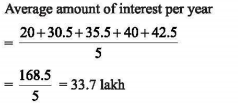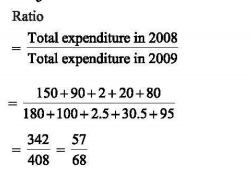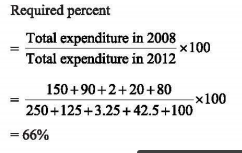# UGC NET PAPER 1 June Shift (Pack of 3) - UGC NET PAPER 1 26th June 2019 Morning Shift

>>>>>>>>UGC NET PAPER 1 26th June 2019 Morning Shift

Read the following comprehension and answer the question.
Consider the following table that shows expenditures of a company (in lakh rupees) per annum
over the given years. Answer the questions based on the data contained in the table:

 Year Salary Transport Bonus Interest on Loans Taxes 2008 150 90 2.00 20.0 80 2009 180 100 2.50 30.5 95 2010 200 110 2.75 35.5 105 2011 240 115 3.00 40.0 85 2012 250 125 3.25 42.5 100

• A

0.9%• B

1.3%• C

1.6%• D

2.0%• Option : B
• Explanation : Total amount of bonus paid by the company
=2 + 2.5 +2.75 +3 + 3.25
= 13.5
Total amount of salary paid by the company
= 150+ 180+200+240+250
= 1020
Required percent = 13.5/1020 X 100 = 1.32%

Read the following comprehension and answer the question.
Consider the following table that shows expenditures of a company (in lakh rupees) per annum
over the given years. Answer the questions based on the data contained in the table:

 Year Salary Transport Bonus Interest on Loans Taxes 2008 150 90 2.00 20.0 80 2009 180 100 2.50 30.5 95 2010 200 110 2.75 35.5 105 2011 240 115 3.00 40.0 85 2012 250 125 3.25 42.5 100

• A

31:40• B

25:36• C

27:30• D

31:36• Option : D
• Explanation : Total expenditure on taxes for all the year
= 80 + 95 + 105 + 85 + 100 = 465
Total expenditure on transport for all the year
= 90 + 100 + 110+ 115 + 125 = 540
Required ratio = 465/540 - 31/36

Read the following comprehension and answer the question.
Consider the following table that shows expenditures of a company (in lakh rupees) per annum
over the given years. Answer the questions based on the data contained in the table:

 Year Salary Transport Bonus Interest on Loans Taxes 2008 150 90 2.00 20.0 80 2009 180 100 2.50 30.5 95 2010 200 110 2.75 35.5 105 2011 240 115 3.00 40.0 85 2012 250 125 3.25 42.5 100

• A

30.5 Lakh• B

32.7 Lakh• C

33.7 Lakh• D

35.5 Lakh• Option : C
• Explanation :Read the following comprehension and answer the question.
Consider the following table that shows expenditures of a company (in lakh rupees) per annum
over the given years. Answer the questions based on the data contained in the table:

 Year Salary Transport Bonus Interest on Loans Taxes 2008 150 90 2.00 20.0 80 2009 180 100 2.50 30.5 95 2010 200 110 2.75 35.5 105 2011 240 115 3.00 40.0 85 2012 250 125 3.25 42.5 100

• A

57:75• B

52:68• C

57:68• D

68:57• Option : C
• Explanation :Read the following comprehension and answer the question.
Consider the following table that shows expenditures of a company (in lakh rupees) per annum
over the given years. Answer the questions based on the data contained in the table:

 Year Salary Transport Bonus Interest on Loans Taxes 2008 150 90 2.00 20.0 80 2009 180 100 2.50 30.5 95 2010 200 110 2.75 35.5 105 2011 240 115 3.00 40.0 85 2012 250 125 3.25 42.5 100

• A

66%• B

69%• C

72%• D

75%• Option : A
• Explanation :